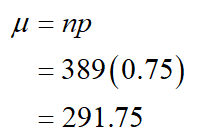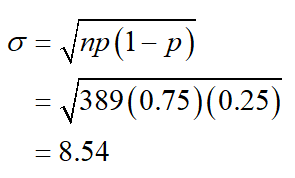# One environmental group did a study of recycling habits in a California community. It found that 75% of the aluminum cans sold in the area were recycled. (Use the normal approximation. Round your answers to four decimal places.)(a) If 389 cans are sold today, what is the probability that 300 or more will be recycled? (b) Of the 389 cans sold, what is the probability that between 260 and 300 will be recycled Please help- I have been working on this for two days!

Question
7 views

One environmental group did a study of recycling habits in a California community. It found that 75% of the aluminum cans sold in the area were recycled. (Use the normal approximation. Round your answers to four decimal places.)

(a) If 389 cans are sold today, what is the probability that 300 or more will be recycled?

(b) Of the 389 cans sold, what is the probability that between 260 and 300 will be recycled

check_circle

star
star
star
star
star
1 Rating
Step 1

(a)

The mean is,Thus, the mean is 291.75.

Standard deviation:Thus, the standard deviation is 8.54.

Step 2

Using correction for continuity error, the probability that 300 or more will be recycled is,Thus, the probability that 300 or more will be recycled is 0.1822.

...

### Want to see the full answer?

See Solution

#### Want to see this answer and more?

Solutions are written by subject experts who are available 24/7. Questions are typically answered within 1 hour.*

See Solution
*Response times may vary by subject and question.
Tagged in